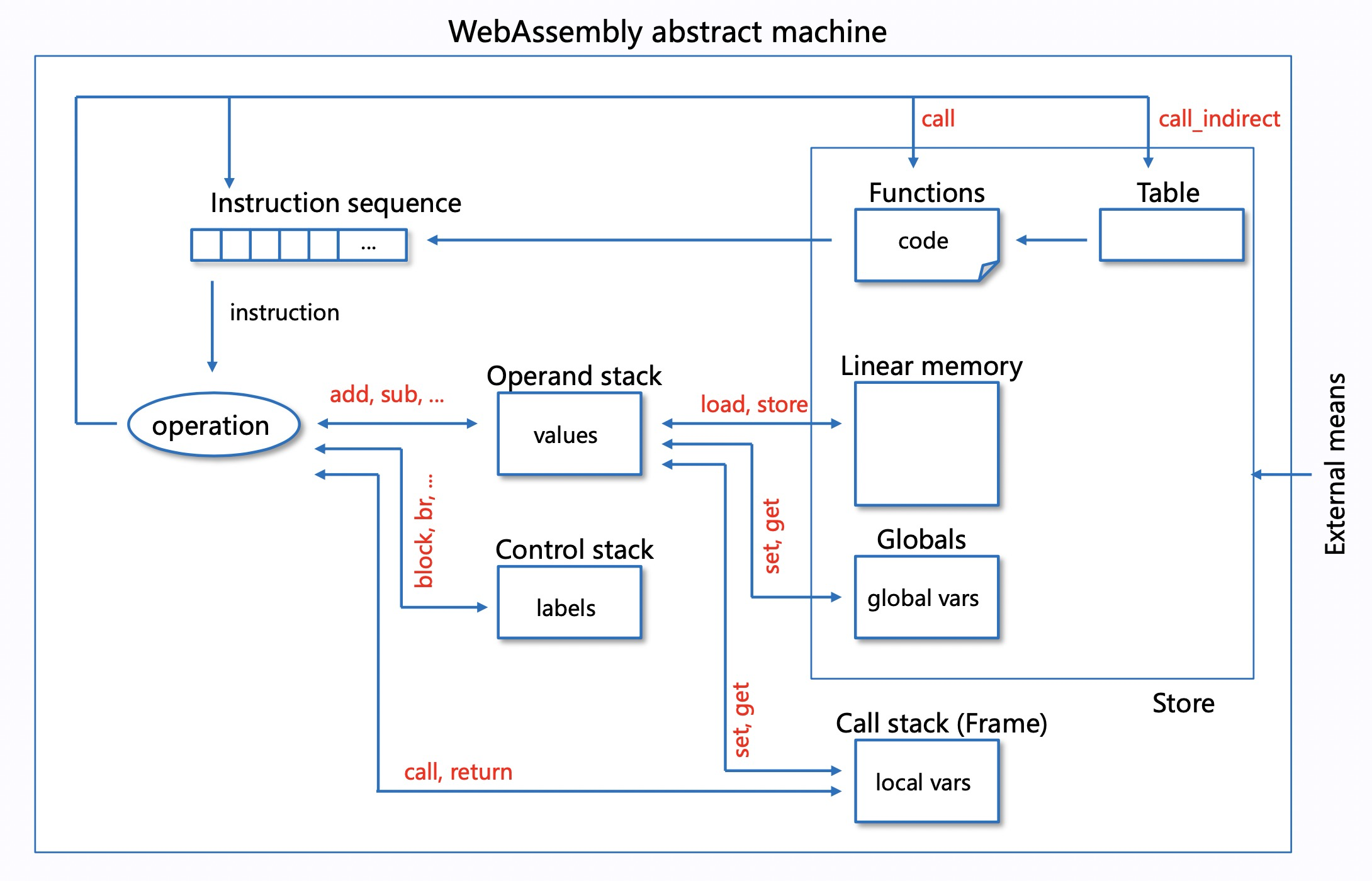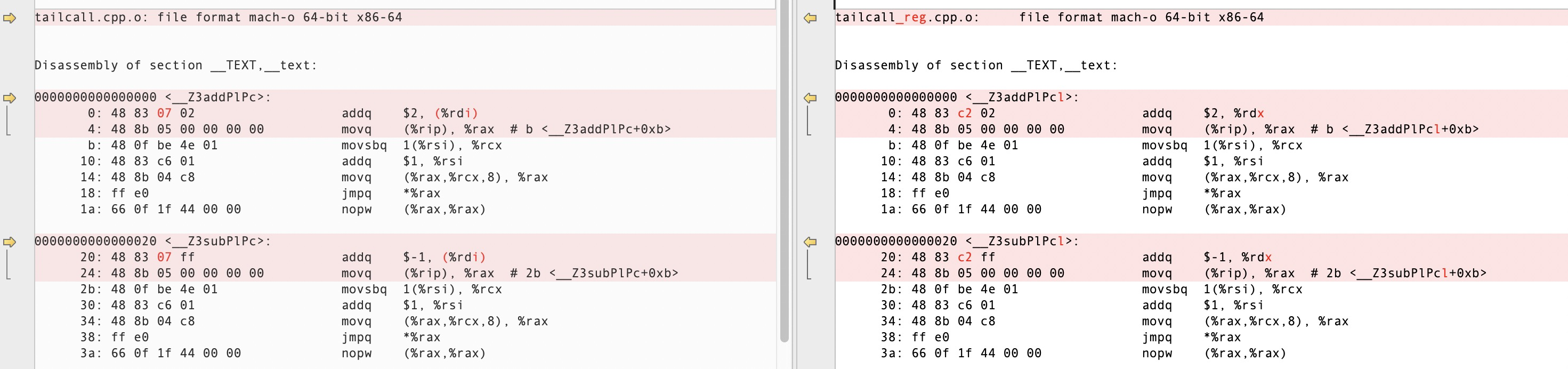# WebAssembly 初探 – Threaded Code

September 19, 2021### 指令分发

``````typedef enum {
SUB,    // *sp -= 1
MUL,    // *sp *= 2
DIV,    // *sp /= 2
END
} OpCode;``````

``````#define CODE_LEN (500000000 * 4)

char *code = new char[CODE_LEN + 1];
for (int i = 0; i < CODE_LEN / 4; i++) {
code[i * 4 + 0] = ADD;
code[i * 4 + 1] = SUB;
code[i * 4 + 2] = MUL;
code[i * 4 + 3] = DIV;
}
code[CODE_LEN] = END;``````

``````long test_switch(char *code) {
long ret = 0;
long *sp = &ret;
char *pc = &code;

while (true) {
switch (*pc++) {
case ADD: *sp += 2; break;
case SUB: *sp -= 1; break;
case MUL: *sp *= 2; break;
case DIV: *sp /= 2; break;
case END: goto END;
default: break;
}
}
END:
return ret;
}``````

``````typedef void (*OpFunc)(long *);

void add(long *sp) { *sp += 2; }
void sub(long *sp) { *sp -= 1; }
void mul(long *sp) { *sp *= 2; }
void div(long *sp) { *sp /= 2; }

long test_call(char *code) {
long ret = 0;
long *sp = &ret;
char *pc = &code;

OpFunc funcs[] = { add, sub, mul, div };
while (*pc != END) {
funcs[*pc++](sp);
}

return ret;
}``````

GCC 支持 Labels as Values (LLVM 也支持)，即可以拿到 label 的地址，得到 void* 类型的值，然后配合 goto，就能运行期动态跳转到不同 label。基于这个特性，虚拟机的指令分发可以有更高效的形式 indirect threading：

``````long test_indirect(char *code) {
long ret = 0;
long *sp = &ret;
char *pc = &code;

void *labels[] = { &&ADD, &&SUB, &&MUL, &&DIV, &&END };
goto *labels[*pc];

*sp += 2; goto *labels[*(++pc)];
SUB:
*sp -= 1; goto *labels[*(++pc)];
MUL:
*sp *= 2; goto *labels[*(++pc)];
DIV:
*sp /= 2; goto *labels[*(++pc)];
END:
return ret;
}``````

### 尾调用

``````typedef void (*OpFunc)(long *, char *);

static OpFunc *funcs = nullptr;
#define NEXT pc++; funcs[*pc](sp, pc);

void add(long *sp, char *pc) { *sp += 2; NEXT }
void sub(long *sp, char *pc) { *sp -= 1; NEXT }
void mul(long *sp, char *pc) { *sp *= 2; NEXT }
void div(long *sp, char *pc) { *sp /= 2; NEXT }
void end(long *sp, char *pc) { }

long test_tailcall(char *code) {
long ret = 0;
long *sp = &ret;
char *pc = &code;

funcs = new OpFunc[]{ add, sub, mul, div, end };
funcs[*pc](sp, pc);

delete[] funcs;
return ret;
}``````

NEXT 宏定义中，我们将 pc++，然后调用下一个指令函数，这样每执行一个指令都会增加一层函数调用栈，指令多了会导致栈溢出，不过由于调用出现在函数最尾部，编译器是可以进行尾调用优化的，即本来应该进行的函数调用相关操作都可以省略(现场已经没用了)，直接跳转到新函数入口地址即可，也即“call”指令优化成了“jmp”指令，从而避免了栈溢出。上面程序如果指定 -O0 优化，运行后会栈溢出报错，指定 -O2 则能正常执行，感兴趣的可以 objdump 看一下优化前后函数生成的指令差异。

### 寄存器

``````typedef void (*OpFunc)(long *, char *, long);

static OpFunc *funcs = nullptr;
#define NEXT pc++; funcs[*pc](sp, pc, reg);

void add(long *sp, char *pc, long reg) { reg += 2; NEXT }
void sub(long *sp, char *pc, long reg) { reg -= 1; NEXT }
void mul(long *sp, char *pc, long reg) { reg *= 2; NEXT }
void div(long *sp, char *pc, long reg) { reg /= 2; NEXT }
void end(long *sp, char *pc, long reg) { *sp = reg; }

long test_tailcall_reg(char *code) {
long ret = 0;
long *sp = &ret;
char *pc = &code;

funcs = new OpFunc[]{ add, sub, mul, div, end };
funcs[*pc](sp, pc, *sp);

delete[] funcs;
return ret;
}``````### 小结

``````switch           2609 ms
indirect         1457 ms
call             2741 ms
tailcall         3184 ms
tailcall_reg     1150 ms``````

``````#if WASM_ENABLE_LABELS_AS_VALUES != 0
#define HANDLE_OPCODE(op) &&HANDLE_##op
DEFINE_GOTO_TABLE (const void*, handle_table);
#undef HANDLE_OPCODE
if (exec_env == NULL) {
global_handle_table = (void **)handle_table;
return;
}
#endif

#if WASM_ENABLE_LABELS_AS_VALUES == 0
while (frame_ip < frame_ip_end) {
opcode = *frame_ip++;